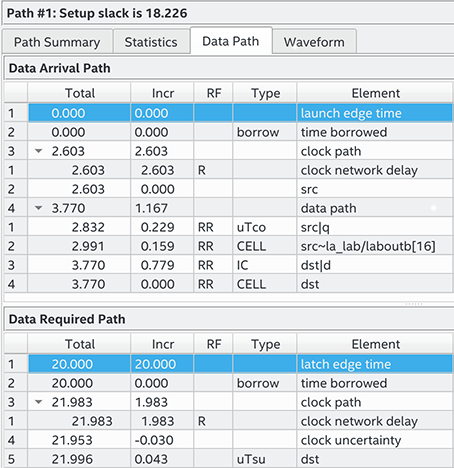ID 683243
Date 8/03/2023
Public

## 3.6.8.5.3. End Multicycle Setup = 2 and End Multicycle Hold = 1

In this example, the end multicycle setup assignment value is two, and the end multicycle hold assignment value is one.

### Multicycle Constraint

set_multicycle_path -from [get_clocks clk_src] -to [get_clocks clk_dst] \
-setup -end 2
set_multicycle_path -from [get_clocks clk_src] -to
\[get_clocks clk_dst] -hold -end 1

In this example, the setup relationship relaxes by one clock period by moving the latching edge to the right of the default latching edge by 1 clock period. The hold relationship relaxes by one clock period by moving the latch edges to the left of the default latching edges by one.

The following shows the setup timing diagram for the analysis that the Timing Analyzer performs:

Figure 124. Setup Timing Diagram
Figure 125. Setup Check Calculation

The most restrictive hold relationship with an end multicycle setup assignment value of two is 20 ns.

The following shows the setup report for this example in the Timing Analyzer and highlights the launch and latch edges.

Figure 126. Setup Report with Setup and Hold Multicycle ExceptionThe following shows the timing diagram for the hold checks for this example. The hold checks are relative to the setup check.

Figure 127. Hold Timing Diagram
Figure 128. Hold Check Calculation

The most restrictive hold relationship with an end multicycle setup assignment value of two and an end multicycle hold assignment value of one is 0 ns.

The following shows the hold report for this example in the Timing Analyzer and highlights the launch and latch edges.

Figure 129. Hold Report with Setup and Hold Multicycle Exception